# April 11, 2019 Name Problem # 1-55 points In the final stages of production, a supply fluid for a...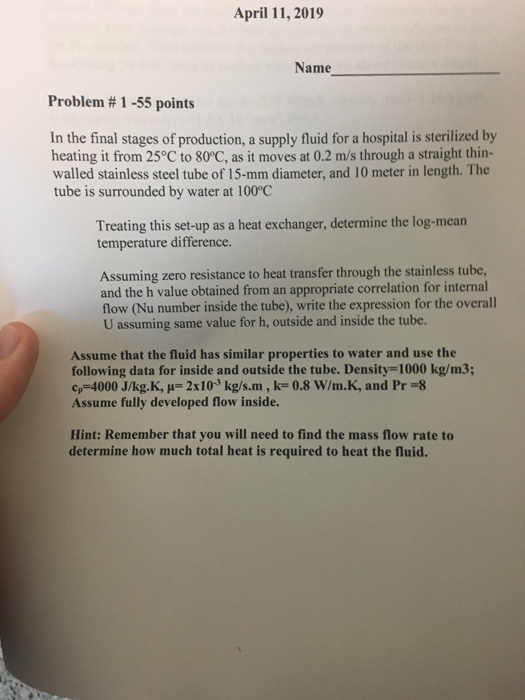April 11, 2019 Name Problem # 1-55 points In the final stages of production, a supply fluid for a hospital is sterilized by heating it from 25°C to 80°C, as it moves at 0.2 m/s through a straight thin- walled stainless steel tube of 15-mm diameter, and 10 meter in length. The tube is surrounded by water at 100°C Treating this set-up as a heat exchanger, determine the log-mean temperature difference Assuming zero resistance to heat transfer through the stainless tube, and the h value obtained from an appropriate correlation for internal flow (Nu number inside the tube), write the expression for the overall U assuming same value for h, outside and inside the tube. Assume that the fluid has similar properties to water and use the following data for inside and outside the tube. Density-1000 kg/m3; cp-4000 J/kg.K, H-2x10 kg/s.m, k- 0.8 W/m.K, and Pr -8 Assume fully developed flow inside. Hint: Remember that you will need to find the mass flow rate to determine how much total heat is required to heat the fluid.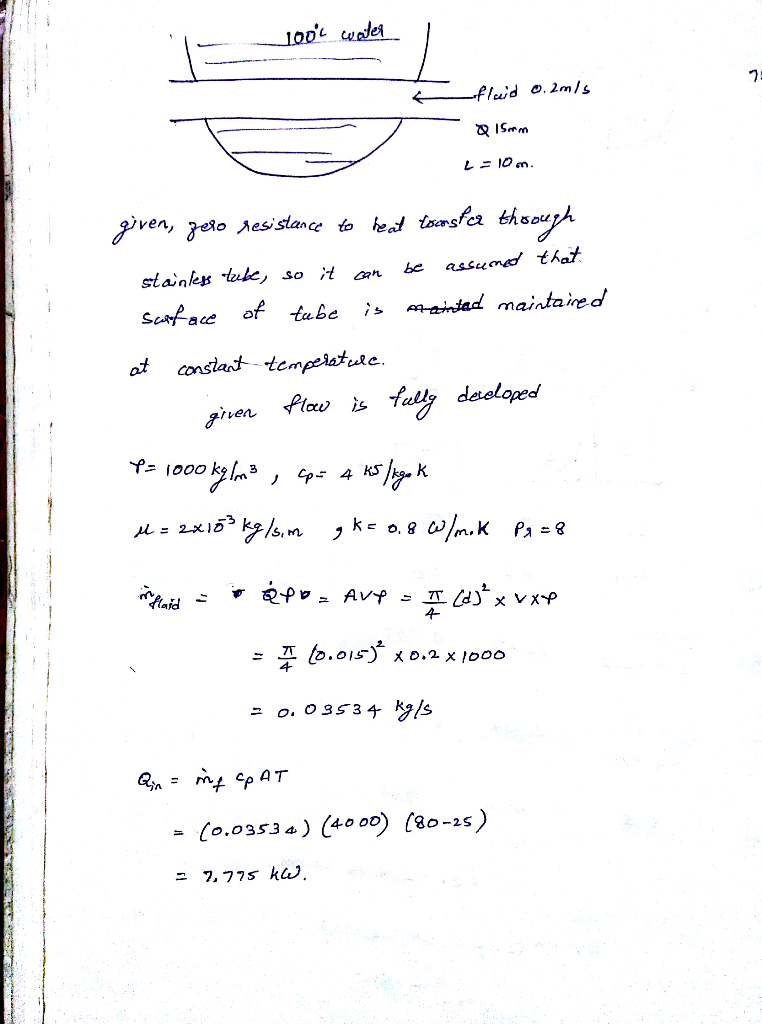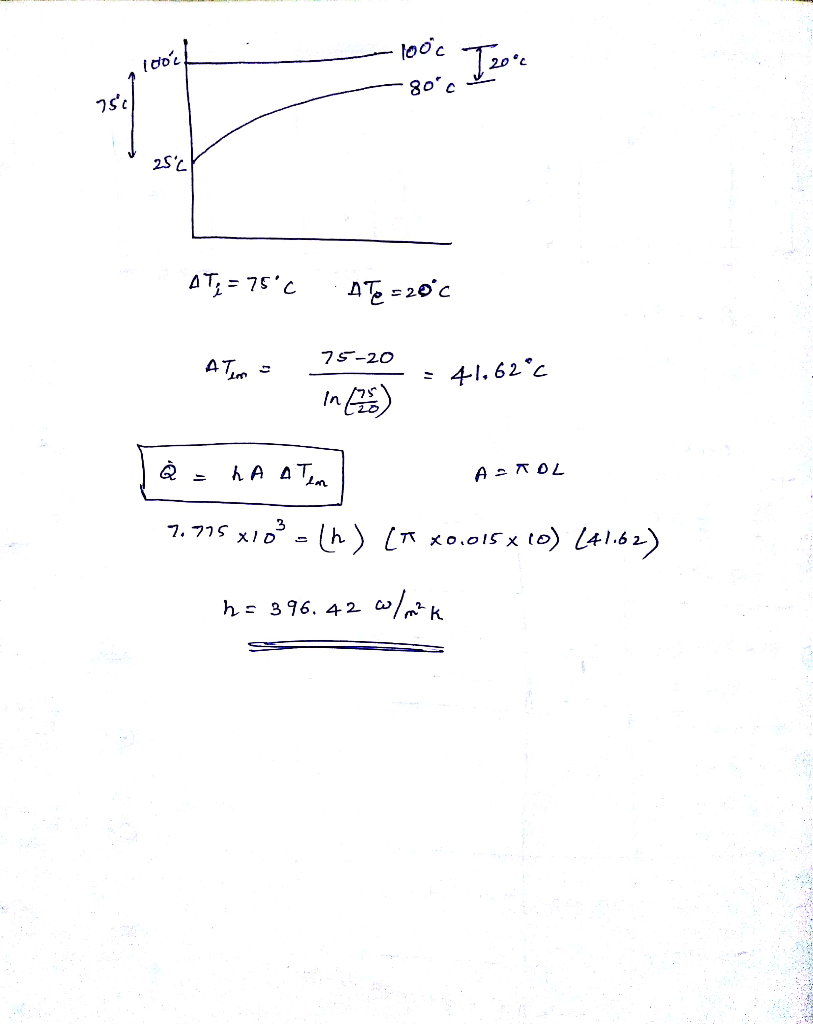##### Add Answer of: April 11, 2019 Name Problem # 1-55 points In the final stages of production, a supply fluid for a...
Similar Homework Help Questions
• ### In the final stages of production, a pharmaceutical is sterilized by heating it from 25 to 75°C a...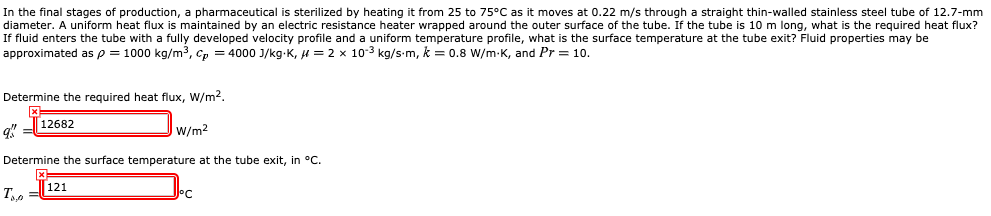In the final stages of production, a pharmaceutical is sterilized by heating it from 25 to 75°C as it moves at 0.22 m/s through a straight thin-walled stainless steel tube of 12.7-mm diameter. A uniform heat flux is maintained by an electric resistance heater wrapped around the outer surface of the tube. If the tube is 10 m long, what is the required heat flux? If fluid enters the tube with a fully developed velocity profile and a uniform temperature...

• ### Problem 4: Heat Exchangers Analysis (25 points) A counterflow plate-type heat exchanger as shown in the...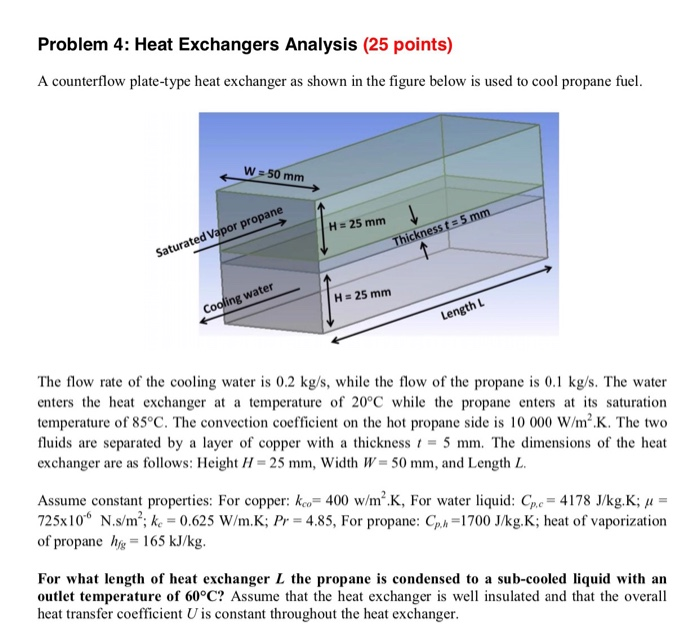Problem 4: Heat Exchangers Analysis (25 points) A counterflow plate-type heat exchanger as shown in the figure below is used to cool propane fuel W 50 mm H 25 mm Saturated Vapor propane Thickness t =5 mm H 25 mm Cooling water Length L The flow rate of the cooling water is 0.2 kg/s, while the flow of the propane is 0.1 kg/s. The water enters the heat exchanger at a temperature of 20°C while the propane enters at its...

• ### Problem 2: Heat exchanger (25 points) Cold water (op 4179 J/kg K) enters the tubes of a heat exchanger at 20 °C at a rate of 3 kgs. while hot oil (cp 2200 J/kg.K) enters the shell at 130 C at the sam...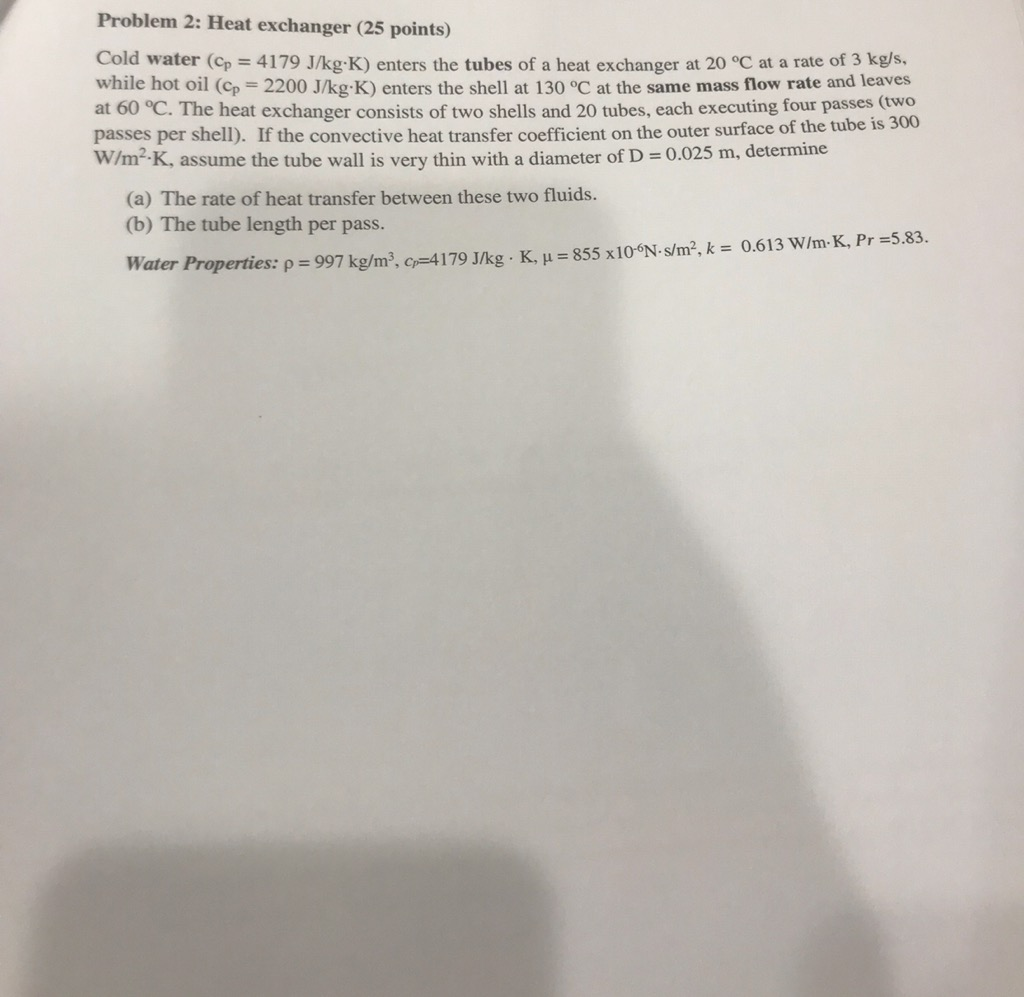Problem 2: Heat exchanger (25 points) Cold water (op 4179 J/kg K) enters the tubes of a heat exchanger at 20 °C at a rate of 3 kgs. while hot oil (cp 2200 J/kg.K) enters the shell at 130 C at the same mass flow rate and leaves at 60°C The heat exchanger consistsoftwo shells and 20 tubes, each executing four passes (two passes per shell). If the W/m2-K, assume the tube wall is very thin with convective heat transfer...

• ### please show me the whole work for question 4) and 5),thank you so much An insulating...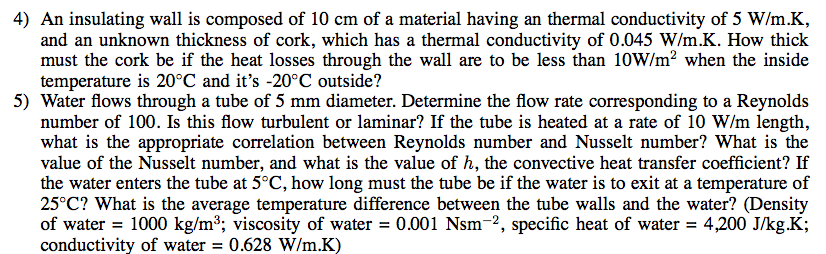please show me the whole work for question 4) and 5),thank you so much An insulating wall is composed of 10 cm of a material having an thermal conductivity of 5 W/m.K, and an unknown thickness of cork, which has a thermal conductivity of 0.045 W/m.K. How thick must the cork be if the heat losses through the wall are to be less than 10W/m2 when the inside temperature is 20degree C and it's -20degree C outside? Water flows through...

• ### A hot fluid passes through a thin-walled tube of 10-mm diameter at 18 kg/h at a...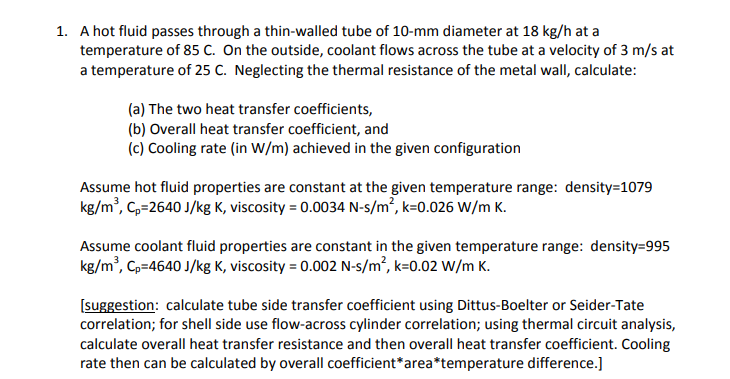A hot fluid passes through a thin-walled tube of 10-mm diameter at 18 kg/h at a temperature of 85 C. On the outside, coolant flows across the tube at a velocity of 3 m/s at a temperature of 25 C. Neglecting the thermal resistance of the metal wall, calculate 1. (a) The two heat transfer coefficients, (b) Overall heat transfer coefficient, and (c) Cooling rate (in W/m) achieved in the given configuration Assume hot fluid properties are constant at the...

• ### Your Initials: Page 5 of 12 Problem 3 (20 pts): Heat exchanger In a supercomputer, signal propagation delays are redaced by resorting to high-density circuit arrangements which are cooled b...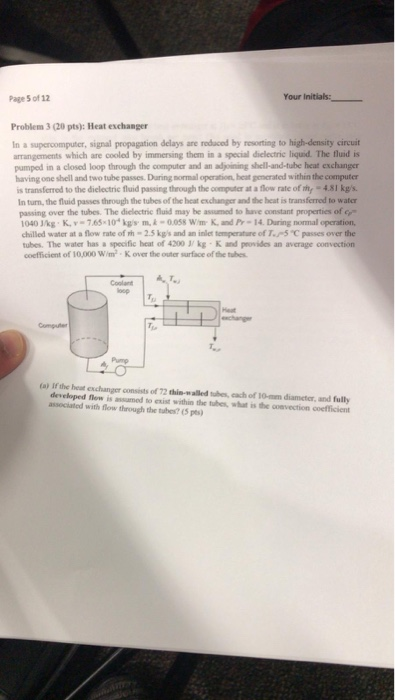Your Initials: Page 5 of 12 Problem 3 (20 pts): Heat exchanger In a supercomputer, signal propagation delays are redaced by resorting to high-density circuit arrangements which are cooled by immersing them in a special dielectric liquid. The fluid is pumped in a closed loop through the computer and an adjoining shell-and-tube heat exchanger having one shell and two tube passes. During mormal operation, heat gencrated within the computer is transferred to the dielectric fluid passing therough the computer at...

• ### Problem 3 (20 points) A cross-flow heat exchanger consists of a bundle of 32 tubes in a long duct. Hot water at 150°C and a mean velocity of 0.5 m/s enters the tubes having inner and outer diameters...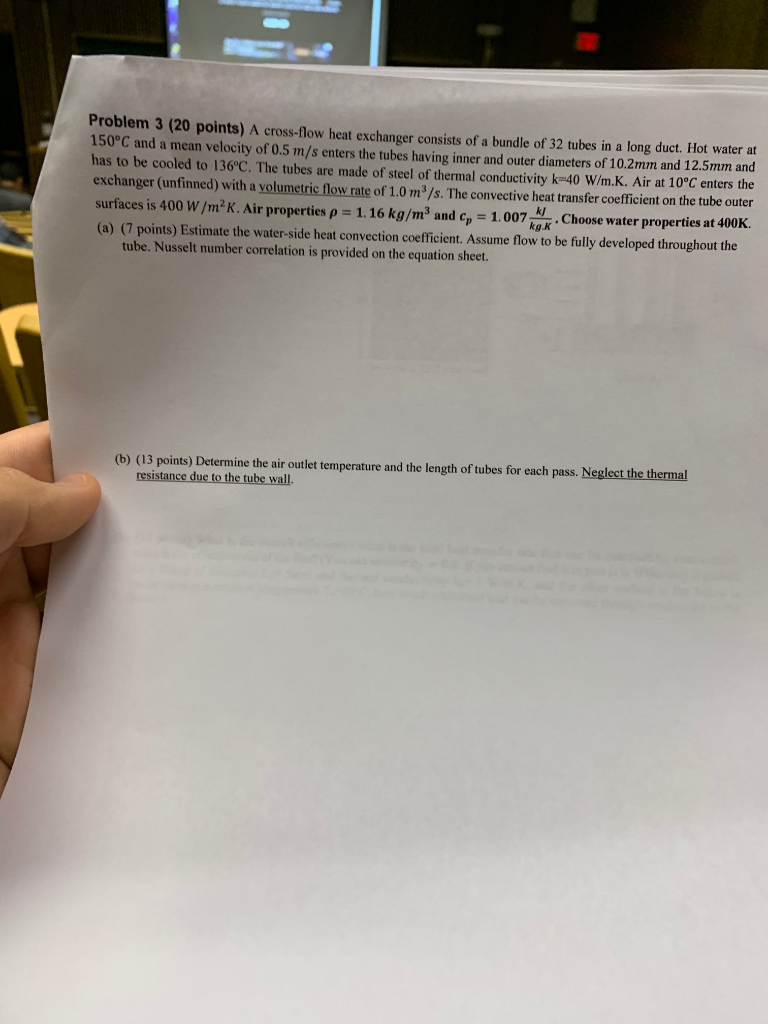Problem 3 (20 points) A cross-flow heat exchanger consists of a bundle of 32 tubes in a long duct. Hot water at 150°C and a mean velocity of 0.5 m/s enters the tubes having inner and outer diameters of 10.2mm and 12.5mm and has to be cooled to 136°C. The tubes are made of steel of thermal conductivity k-40 W/m.K. Air at 10°c enters the exchanger (unfinned) with a volumetric flow rate of 1.0 m3/s. The convective heat transfer coefficient...

• ### PROBLEM #3 (30 points) A shell-and (N 20) is used to heat vegetable oil on the shell side using hot water on tethat side. is Lp- 3.0 m). Each copper tube has dimensions of 15.56-mm ID and 19.05-...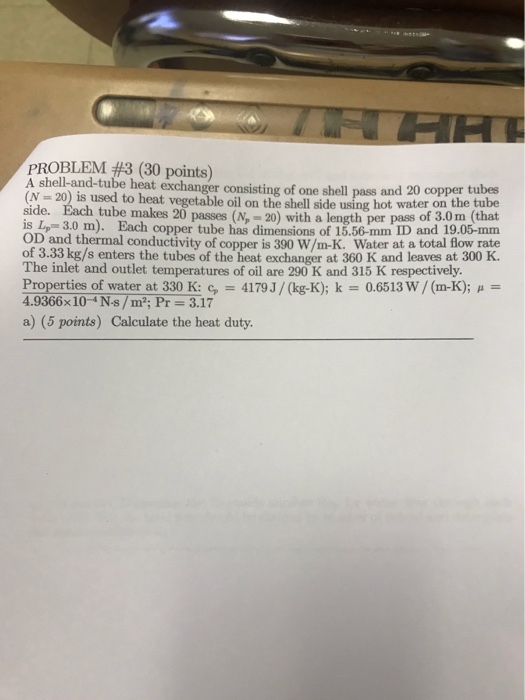PROBLEM #3 (30 points) A shell-and (N 20) is used to heat vegetable oil on the shell side using hot water on tethat side. is Lp- 3.0 m). Each copper tube has dimensions of 15.56-mm ID and 19.05-mm OD and thermal conductivity of copper is 390 W/m-K. Water at a total flow rate of 3.33 kg/s enters the tubes of the heat exchanger at 360 K and leaves at 300 K The inlet and outlet temperatures of oil are 290...

• ### 25 PRR HEAT TRANSFER NAME: UNIT SIX AND SEVEN EXCHANGERS BOILING AND CONDENSATION, He- Solve the following problems:...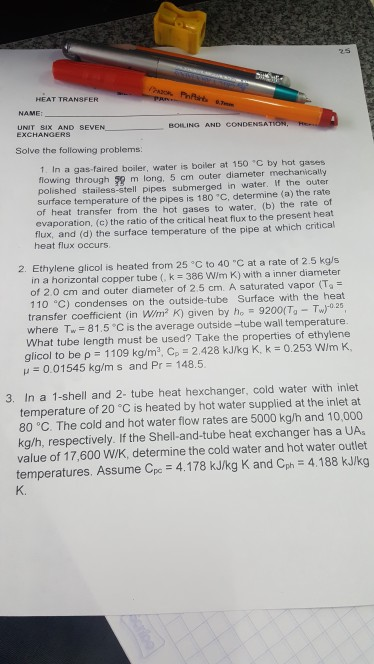25 PRR HEAT TRANSFER NAME: UNIT SIX AND SEVEN EXCHANGERS BOILING AND CONDENSATION, He- Solve the following problems: 1. In a gas-faired boiler, water is boiler at 150 C by hot gases flowing through 59 m long, 5 cm outer diameter mechanically polished stailess-stell pipes submerged in water, If the outer surface temperature of the pipes is 180 °C. determine (a) the rate of heat transfer from the hot gases to water. (b) the rate of evaporation, (c) the ratio...

• ### 1 CPD4701 Assignment 2/2019 Question 2 shell and-tube heat exchanger was designed for the following service: Cold strea...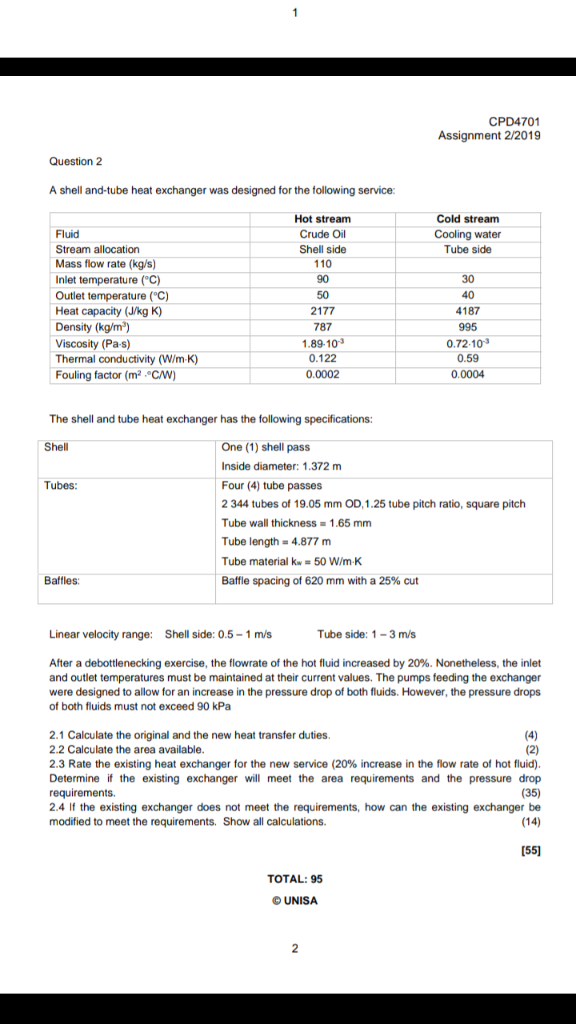1 CPD4701 Assignment 2/2019 Question 2 shell and-tube heat exchanger was designed for the following service: Cold stream Hot stream Crude Oil Fluid Cooling water Tube side Stream allocation Shell side Mass flow rate (kg/s) 110 30 Inlet temperature (C) 90 Outlet temperature (C) Heat capacity (J/kg K) Density (kg/m2) Viscosity (Pa-s) Thermal conductivity (W/m-K) Fouling factor (m2 CW) 40 50 2177 4187 787 995 0.72-10 1.89-103 0.122 0.59 0.0002 0.0004 The shell and tube heat exchanger has the following...

Need Online Homework Help?